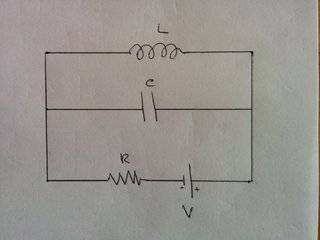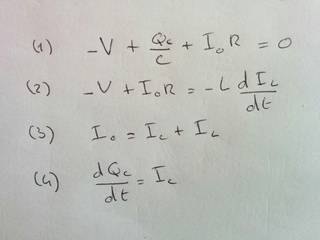Solving a parallel LC circuit with DC current

Take the following circuit (capacitor initially not charged) :I am trying to solve for the current everywhere as a function of time. I set up the following equations (are they right ?) :Then, any way I try it, I end up with some messy integrals. Even using wolfram alpha to get those integrals solutions, I can't find out I_0 (current in the bottom wire with the battery), I_C (current in the middle wire with the capacitor), and I_L (current in the upper wire with the inductor) as functions of time.

I suspect it shouldn't be that messy, I must miss something somewhere. Can anyone solve this for me?

I understand that :

- at first (t = 0, battery just plugged) the current must be 0 in the inductor (upper wire) and equal to VR in the middle and lower wires
- after a long time (t = infinity), current must be 0 in the capacitor (middle wire) and equal to VR in the inductor (upper wire) and in the resistance (lower wire)

What I am trying to understand is what happens in between t = 0 and t = infinity.

Hesch
Gold Member
Do you know about Laplace transforms?

The impedance in the upper wire will be: sL.
In the middle wire: 1/sC.
In the lower wire: R.

Now, sketch a model of the circuit and solve the transfer functions as for the currents, when the battery is connected.
What I am trying to understand is what happens in between t = 0 and t = infinity.
The currents through upper and middle wire will oscillate.

The input function when you switch on the the battery will be: V/s. ( step function ).

Last edited:
vela
Staff Emeritus
Homework Helper
It would help if you drew in the currents and labeled the potential drops across each element.

If you differentiate (1) and use (4), you get
$$\frac{dI_0}{dt} = \frac{1}{RC} I_C.$$ Then use (3) to eliminate ##I_C## to get
$$\frac{dI_0}{dt} = \frac{1}{RC} I_0 - \frac{1}{RC} I_L.$$ Rewriting (2) slightly gives
$$\frac{dI_L}{dt} = \frac VL - \frac RL I_0.$$ You can solve this system using matrix methods. Alternately, you can solve the bottom equation for ##I_0## and then substitute the result into the second equation to get a second-order differential equation for ##I_L##.

Do you know about Laplace transforms?

I don't, sadly. Is it really necessary here?

The impedance in the upper wire will be: sL.
In the middle wire: 1/sC.

Hmm, that looks like what I know, if you refer to s as the driving frequency. However, the voltage source here is DC, not AC.

The currents through upper and middle wire will oscillate.

Are you sure? This is DC current. One thing seems pretty sure, at t = infinity, nothing oscillates. Do you mean there are oscillations before that time? Why? My guess would have been that the current trough the capacitor decreases continuously while it increases in the inductor.

You can solve this system using matrix methods. Alternately, you can solve the bottom equation for I0I_0 and then substitute the result into the second equation to get a second-order differential equation for ILI_L.

Thanks. Sadly I don't know yet how to solve 2nd order differential equations. Do the matrix methods you refer need linear algebra? I don't know those as well. But nice to get the right equation to solve!

Hesch
Gold Member
But anyway:
Hmm, that looks like what I know, if you refer to s as the driving frequency. However, the voltage source here is DC, not AC.
S is not a driving frequency. Say you have a time function: f(t). If you Laplace transform it to f(s). Then:

L(df(t)/dt) = s*f(s)

and

L( f(t)dt ) = f(s)/s
Are you sure? This is DC current.
Yes I am. A LC-connenction will oscillate by any disturbance, such as beeing connected to a battery.
at t = infinity, nothing oscillates.
You are right because also the voltage across the LC will oscillate, thus giving some losses in the resistor. But if you disconnect the resistor just after the oscillation has started, the remaining LC-circuit will in principle oscillate forever, because no resistance is included in the coil.
My guess would have been that the current trough the capacitor decreases continuously while it increases in the inductor.
No, the energy will by turns be stored in L ( E = ½*L*I2 ) or in C ( E = ½*V2*C ). The current/voltage will overshoot all the time.

•DoobleD
Thank you for the answers, and sorry for the delay.

Yes I am. A LC-connenction will oscillate by any disturbance, such as beeing connected to a battery.

Ok. I kind of instinctively, and incorrectly, thought it was different with DC.

You are right because also the voltage across the LC will oscillate, thus giving some losses in the resistor. But if you disconnect the resistor just after the oscillation has started, the remaining LC-circuit will in principle oscillate forever, because no resistance is included in the coil.

Ok. It's really hard to picture. With simple LC or RLC circuits in series, it's easier to understand. Is there some intuitive way to understand this ?

Some of my main interrogations are :

When does the oscillation starts ? Right after current starts to flow, inductance prevents current in the upper part, so does the oscillation really starts just after current is prevented, right when some of it starts to flow through the inductor ?
How does the battery reacts to this oscillation ? Does it recharge/discharge at each oscillation, while always discharging a little more at each cycle due to heat dissipated by R ?

NascentOxygen
Staff Emeritus
•